←  Back to Time Tracking Library

# Project profitability: How much is enough?

Last updated: May 31, 2022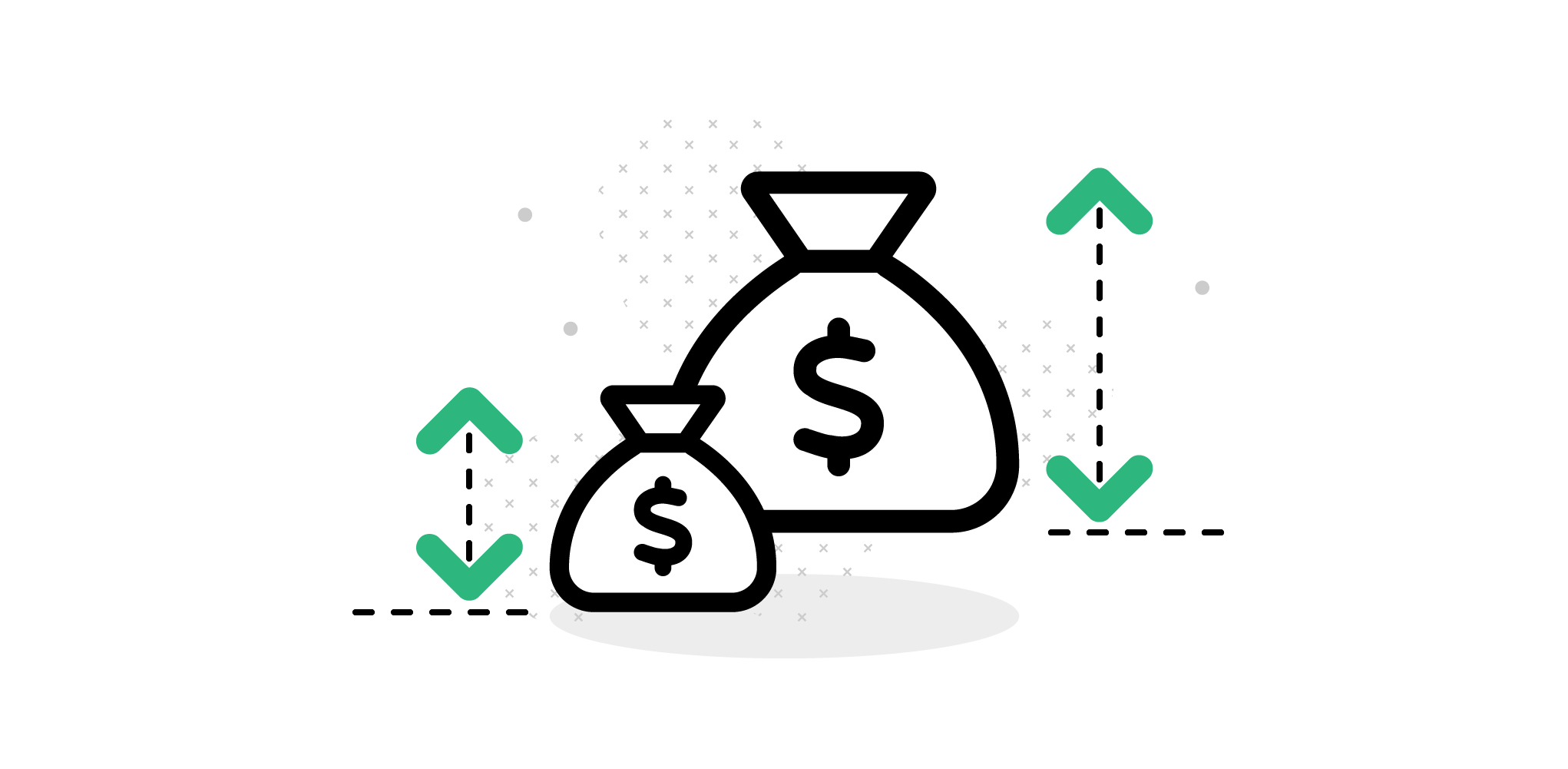All projects should be profitable, but how profitable? And is the project with the highest return on investment always the right choice? Answering these questions requires a nuanced understanding of risk and return. With careful modeling, however, it is possible to go into an uncertain future prepared, and optimize limited capital to get the best results for you and your shareholders.

The aim of every project should be to achieve the highest possible profitability. As we have covered in a separate article, this can be achieved by properly estimating effort, pricing accordingly, and monitoring progress closely. In other words, let data - past and present - guide decision-making.

Corporate finance departments have evolved a number of alternative tools to help them decide whether to enter new projects, ranging from rules-of-thumb to highly nuanced methodologies that rely on detailed models.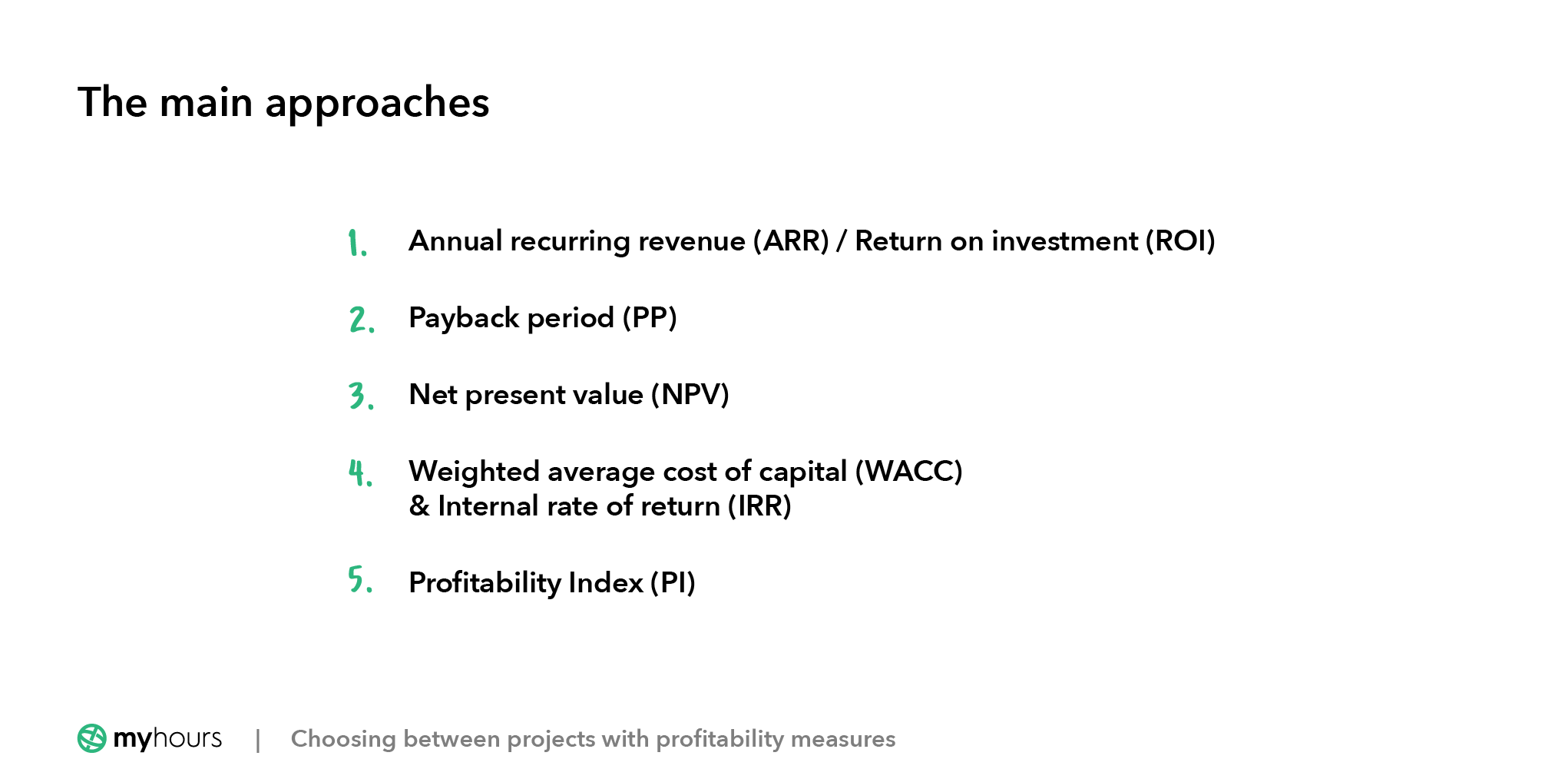• Annual recurring revenue (ARR) / Return on investment (ROI)

• Payback period (PP)

• Net present value (NPV)

• Weighted average cost of capital (WACC) & Internal rate of return (IRR)

• Profitability Index (PI)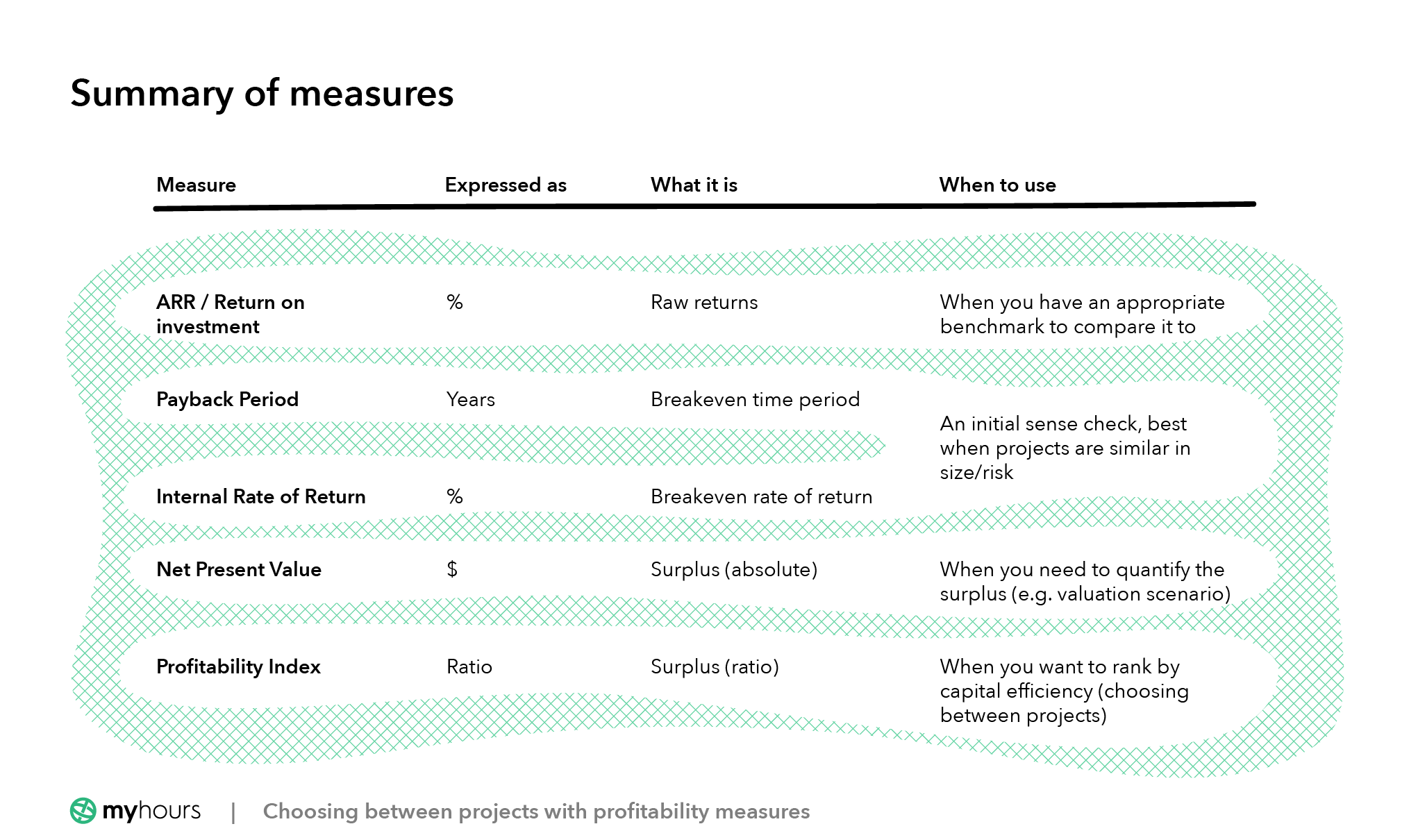## Basics: What’s the big deal?

At a basic level, profit is equal to revenues minus costs. In the case of a short project, this is relatively simple to calculate: project fees minus manpower costs and other expenses incurred.

### A simple illustration

Using our eight-week strategic review as an example, the calculation could be: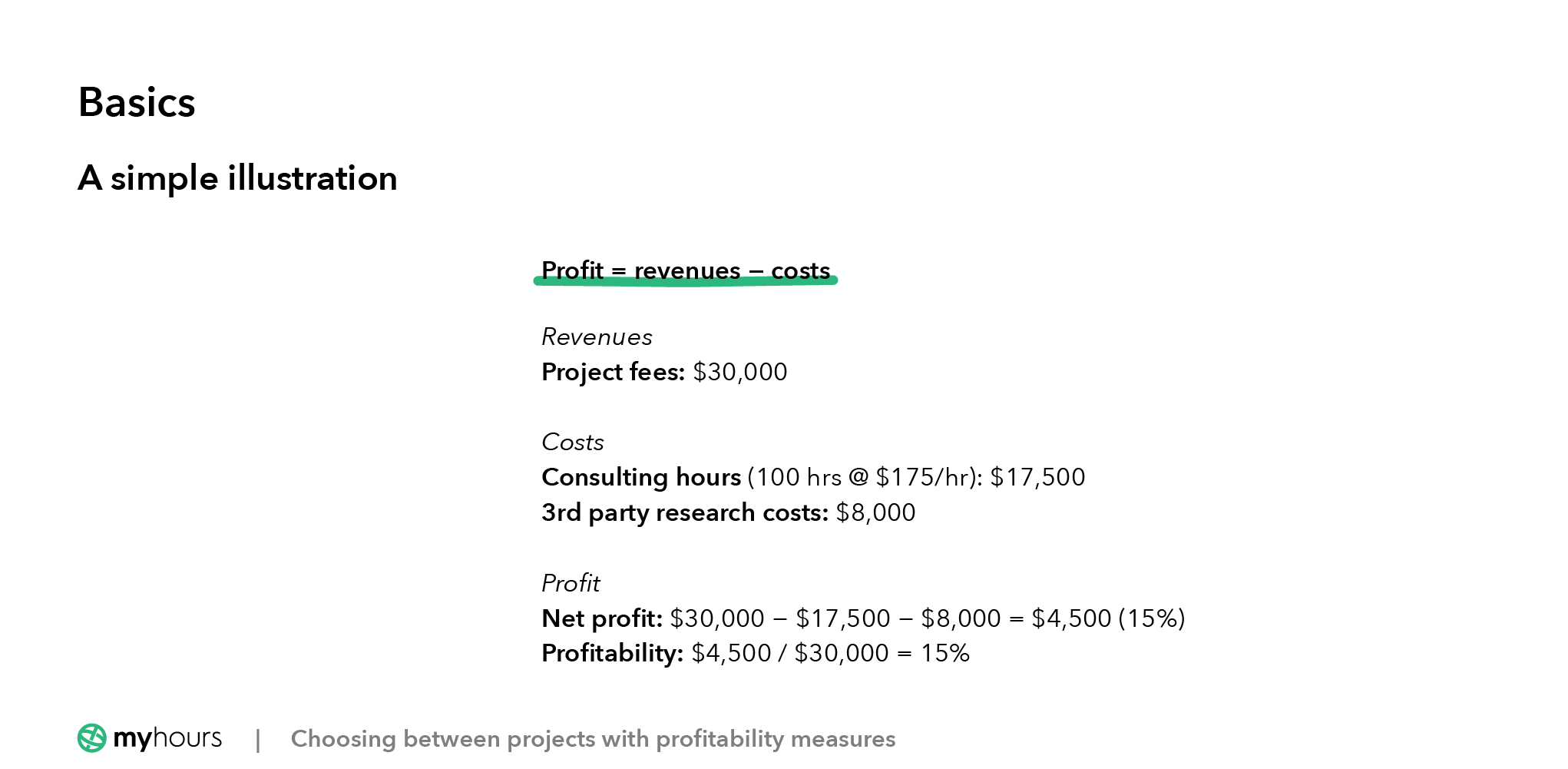Since all of these numbers fall within the same eight weeks (and the same accounting period), calculating profit and profitability is straightforward.

Another way of thinking about profitability, however, is of return on investment - how much you get from the resources and/or capital you commit to something.

### Return on Investment (ROI)

Return on Investment (ROI) is also known as Accounting Rate of Return (ARR). It expresses how much you get back compared to what you put in. An example of ROI would be the interest rate on a savings account, which you can use to compare different banks and savings products.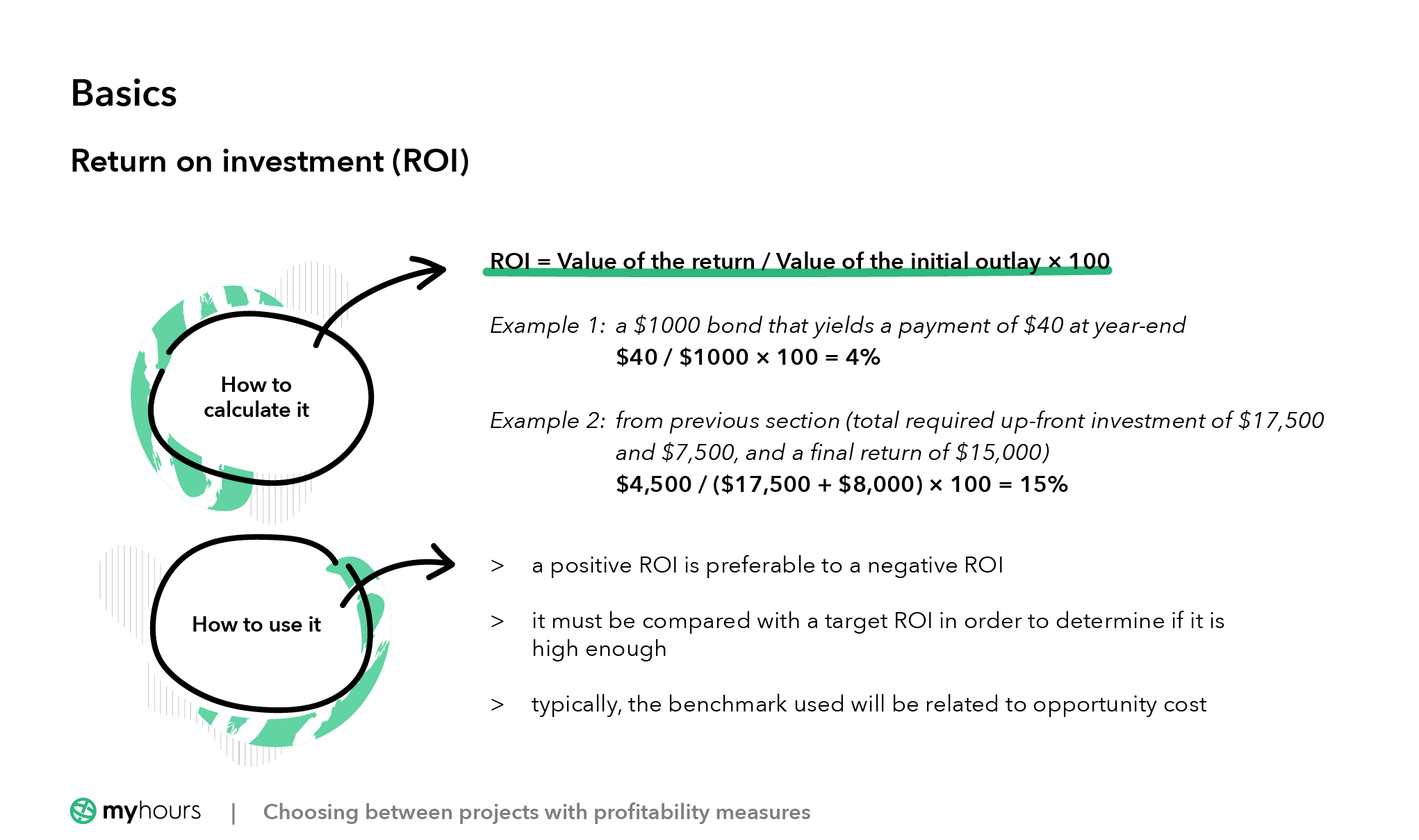#### How to calculate it

ROI is one of the simplest and most intuitive methods for evaluating a course of action. It is expressed as a percentage, and calculated as follows:

ROI = Value of the Return / Value of the Initial Outlay * 100

One example would be a \$1000 bond that yields a payment of \$40 at year-end:

- \$40 / \$1000 * 100 = 4%

To take the example in the previous section: given total required up-front investment of \$17,500 and \$7,500, and a final return of \$15,000:

- \$4,500 / (\$17,500 + \$8,000) * 100 = 15%

#### How to use it

In the context of a business that generates revenues from people leveraging their time, one has a constraint on resources (one person cannot do two full-time projects simultaneously). In other words, one must consider opportunity cost.

To continue the example of the eight-week project above, if another client were to appear requesting a similar piece of work for a higher price, the best business decision (all other things being equal) would be to prioritize the higher-paying project, and generate a higher return on ‘capital’ (in this case, the cost to maintain the team).

Clearly, a positive ROI is preferable to a negative ROI. Beyond this, however, it is difficult to use ROI in isolation. It must be compared with something - a target ROI - in order to determine if it is high enough. Typically, the benchmark used will be related to opportunity cost, as we will see further below.

## Dealing with more complex decisions

What about projects that are not neatly self-contained within a few months or a calendar year? What about projects where, for example:

• There is a large, initial financial outlay required

• There is a long delay before revenues appear

• Revenues last for a number of years into the future

Consider the following trajectory of net cash flow (total \$ out - total \$ in). Should you take this project on?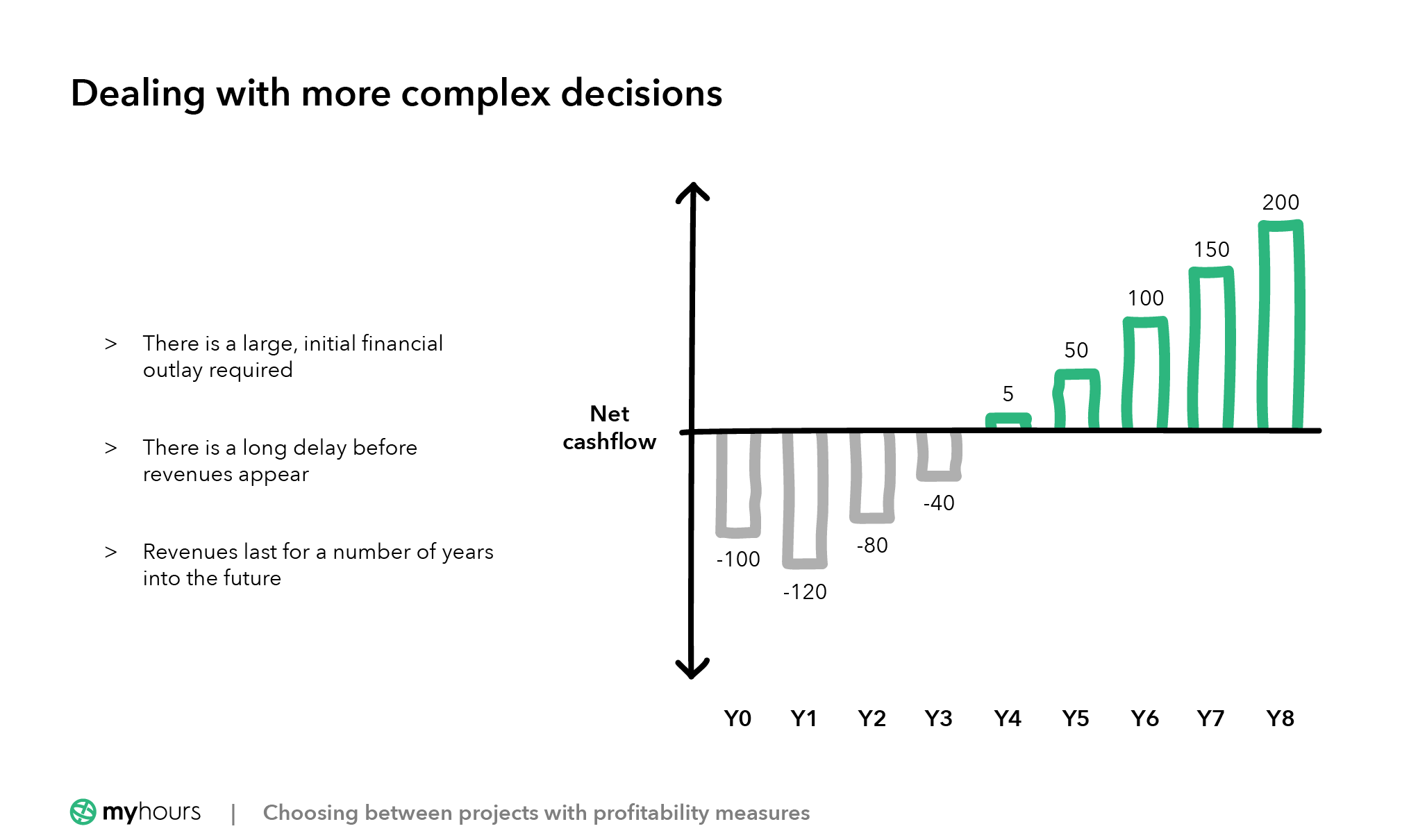Answering this question requires you to perform some kind of calculation that allows you to decide how profitable the project is in comparison to others. The point of comparability is important because, when capital is constrained, it is not possible to take on an infinite number of projects, even if they are all profitable.

### Payback Period (PB)

The Payback method answers the question, asking, “How long until I get my initial investment back?”. Like other measures, it generates a number - in this case, in years - that can be compared easily across projects of differing size and return.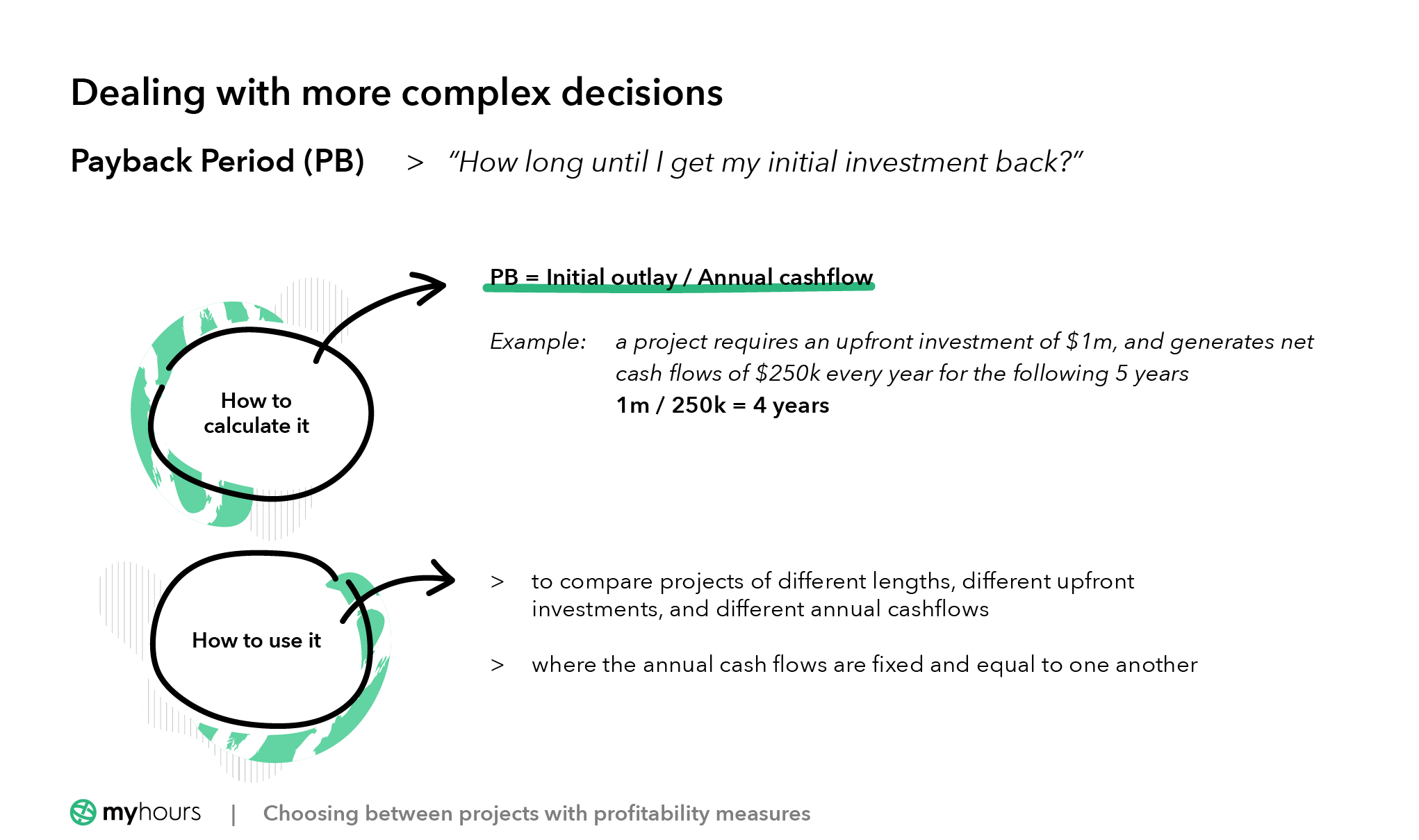#### How to calculate it

Suppose a project requires an upfront investment of \$1m, and generates net cash flows of \$250k every year for the following 5 years. After 4 years (4 * 250k) the project would have ‘paid back’ its original investment.

One calculates the Payback Period as follows:

PB = Initial outlay / Annual cashflow

So in this case: 1m / 250k = 4 years. If the annual cash inflow was projected to be \$400k, the payback period would be shorter (1m / 400k = 2.5 years).

#### How to use it

Using the Payback method definitely falls into the ‘rule of thumb’ category.

The method is quick and allows you to compare projects of different lengths, different upfront investments, and different annual cashflows. In general, a faster payback period is preferable, as it allows capital to be redeployed on other projects.

The Payback method only works, however, in cases where the annual cash flows are fixed and equal to one another (rare in real life).

It also assumes that speed of recouping the investment is the only factor. This ignores the fact that a project might deliver a higher return, or be less risky. It also takes no account of the time value of money - \$250k in Year 4 is not equal to \$250k in Year 2.

Making capital allocation decisions solely on the basis of the payback method, therefore, is unwise. This is particularly true in cases where the projects are materially different from one another in any of the above-mentioned respects.

### Net Present Value (NPV)

NPV is based on the idea that the value of an enterprise is equal to the discounted sum of all future cash flows. We’ll explain what this means further down! But basically, a project has a positive NPV if the cash flows it generates exceed the initial investment (and vice versa).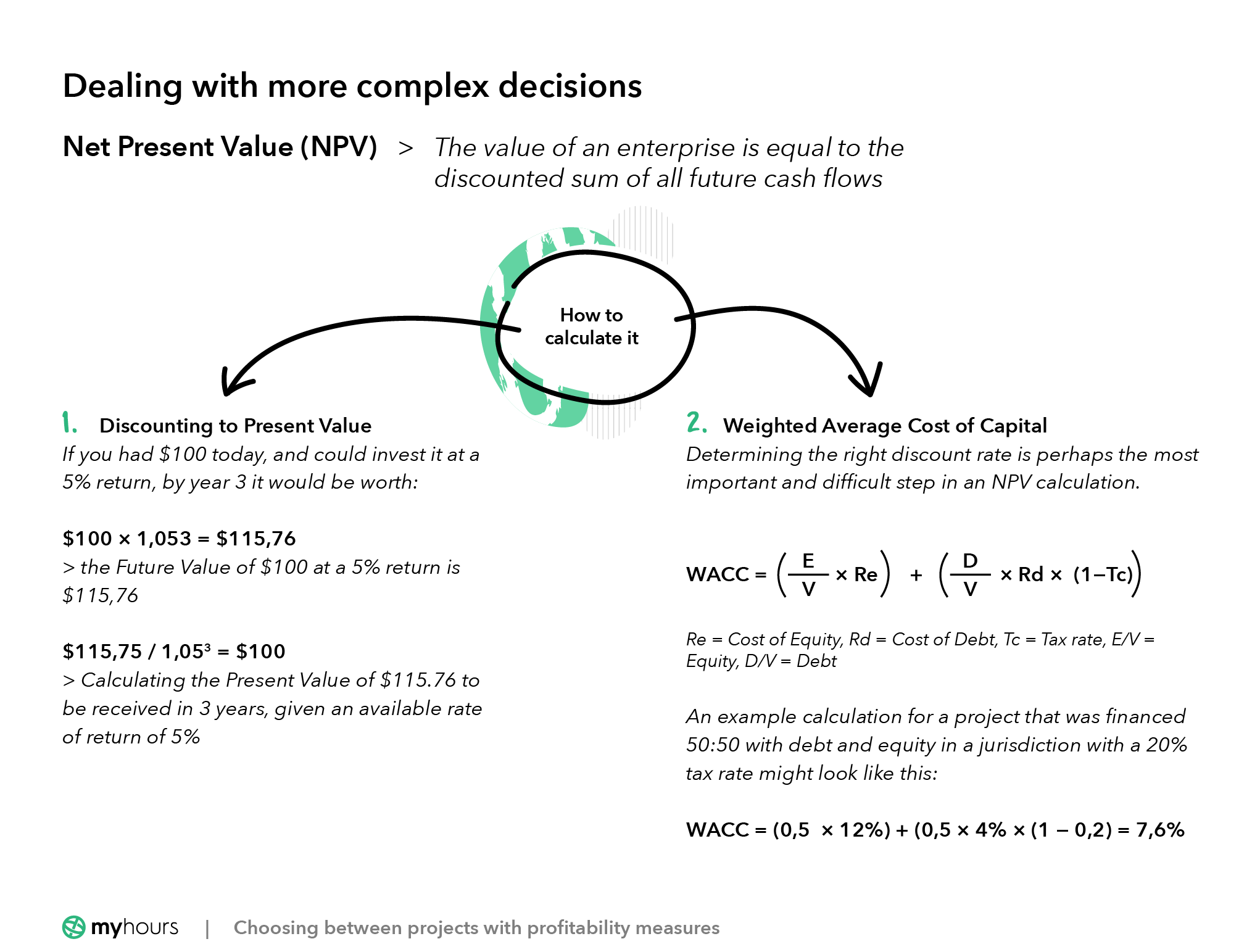#### How to calculate it

Net Present Value is a mainstay not only of project evaluation (whether to take on a project) but also of company valuation (how much to pay for a company).

Take the example of the chart above. It is hard to determine the profitability at a glance, because the cashflows all occur in different periods. In our basic example, all the numbers occurred in the same period, and we could simply add up the revenues and subtract the costs.

NPV converts all future cash flows to their present value (i.e. what they are worth today), thus making them comparable. It adds these different values together - both the negative and the positive - and hence net.

Discounting to Present Value
How is it possible to convert a future value (say in Year 3) so that it is comparable with a number in Year 1? The easiest way to understand this is in reverse.

If you had \$100 today, and could invest it at a 5% return, by year 3 it would be worth: \$100 * 1.05^3 = \$115.76.

In other words, the Future Value of \$100 at a 5% return is \$115.76.

So, if someone asked you to calculate the Present Value of \$115.76 to be received in 3 years, given an available rate of return of 5%, what would you do? Simply, perform the calculation in reverse. \$115.75 / 1.05^3 = \$100.

This process is called discounting, and as you have seen, it is simply the process of compounding, but reversed. The % rate used is known as the discount rate.

Weighted” Average Cost of Capital (WACC)

Cost of Capital’ is another way of saying the Return on Investment that an investor in a project of equivalent risk would expect to receive. For a project that is financed with a mixture of equity and debt, as many projects are, the correct discount rate is referred to as the “Weighted” Average Cost of Capital, as it reflects the relative weight of the debt and equity components.

Determining the right discount rate is perhaps the most important - and difficult - step in an NPV calculation.

Here is the formula - don’t worry if you don’t understand it completely!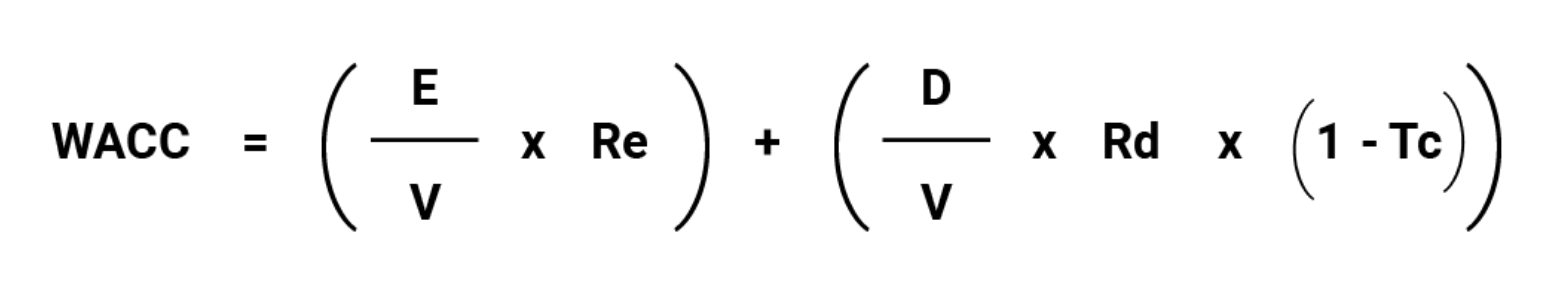Re is the Cost of Equity, or the required rate of return for an equity holder in a similarly risky project. Meanwhile, Rd is the Cost of Debt, or the return that a debt holder would expect.

The Cost of Debt is reduced by the Tax rate (Tc), because interest expenses are tax-deductible.

The final figure is ‘weighted’ because the two rates of return (for equity and debt) are weighted in proportion to the overall level of Equity (E/V) and Debt (D/V) as a portion of the total capital.

An example calculation for a project that was financed 50:50 with debt and equity in a jurisdiction with a 20% tax rate might look like this:

WACC = (0.5  * 12%) + (0.5 * 4% * (1 - 0.2) = 7.6%

Calculating the cost of equity is another level of detail, but you are unlikely to need to do this unless you are an investment banker. The above should give you enough working knowledge to be going on with.

#### How to use it

The WACC, therefore, is the same rate of return that investors could reasonably expect to achieve from a similar project, and should be used to calculate the present value of its cash flows.

This required rate of return will correlate with risk. To take a simple example, here are two projects that deliver a payoff within 3 years.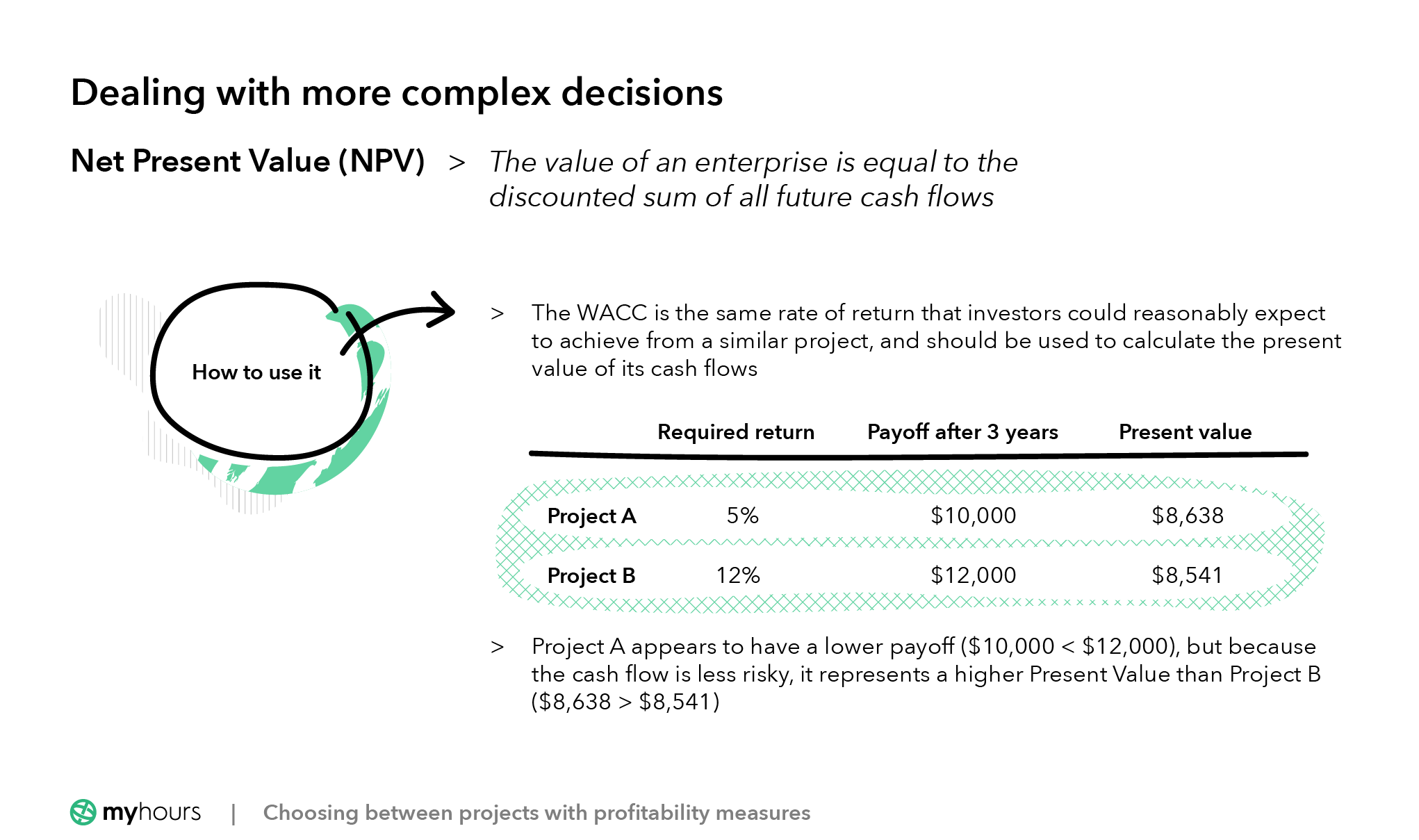Project A appears to have a lower payoff (\$10,000 < \$12,000), but because the cash flow is less risky, it represents a higher Present Value than Project B (\$8,638 > \$8,541).

Calculating and comparing NPV between projects is much more complex in practice, as cash flows are spread across multiple years, and may (in the case of a company) continue indefinitely. This is not an article on valuation, however, and the above provides enough detail for most projects.

There are additional measures that are related to and based on the NPV methodology, including the Internal Rate of Return (IRR) and the Profitability Index (PI), which we will examine next.

### Internal Rate of Return (IRR)

The definition of the Internal Rate of Return is quite short: it is the discount rate at which the NPV for a given set of cash flows is zero. Another way of saying this is the discount rate at which the future cash flows is equal to the upfront investment (hence they cancel one another out).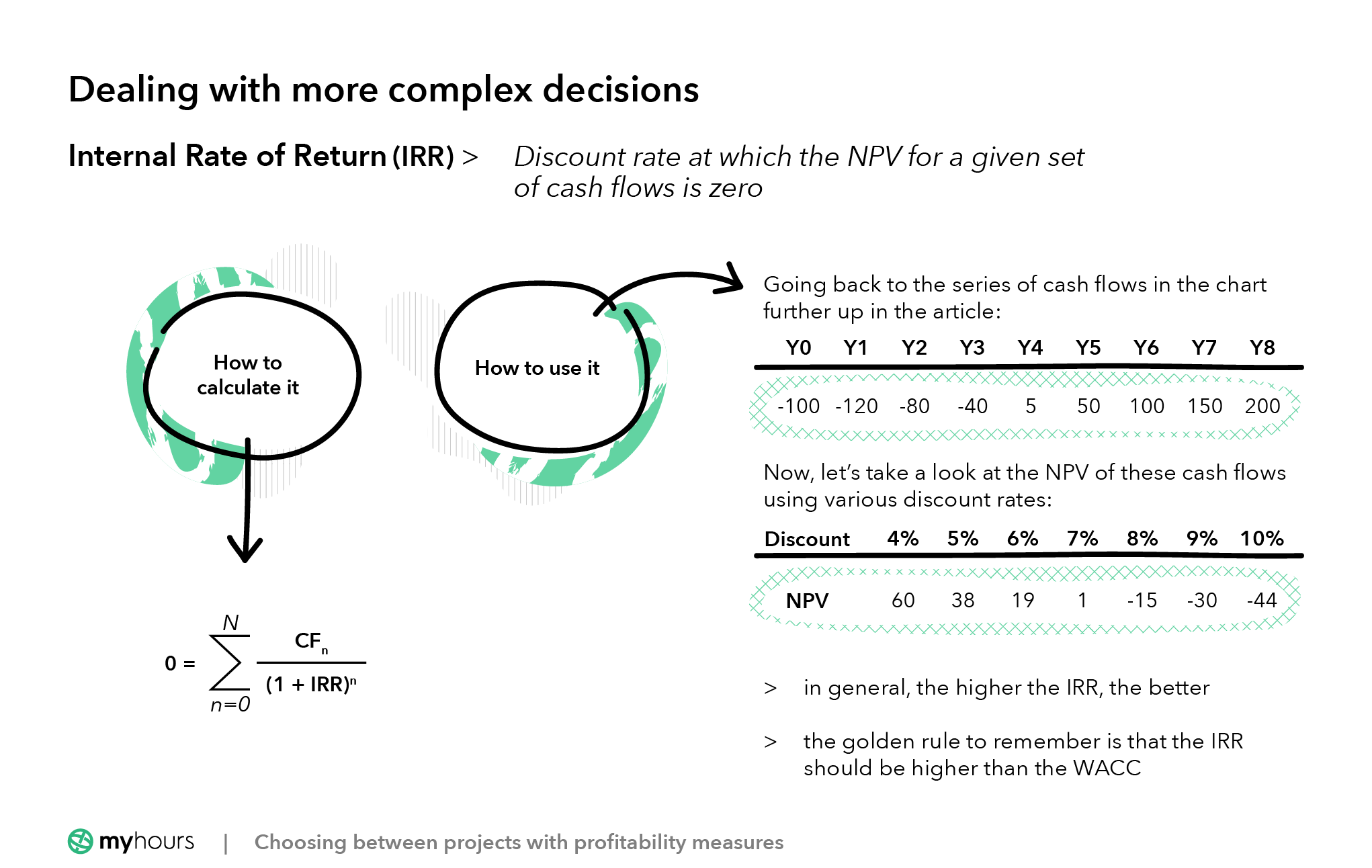#### How to calculate it

Mathematically, the IRR calculation looks like this: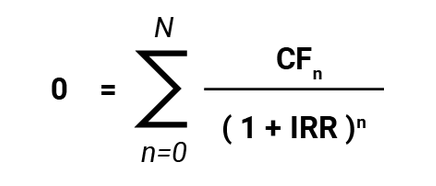While apparently simple, the concept of the IRR isn’t particularly easy to understand, nor what it is used for! So let’s take an example.

#### How to use it

Going back to the series of cash flows in the chart further up in the article: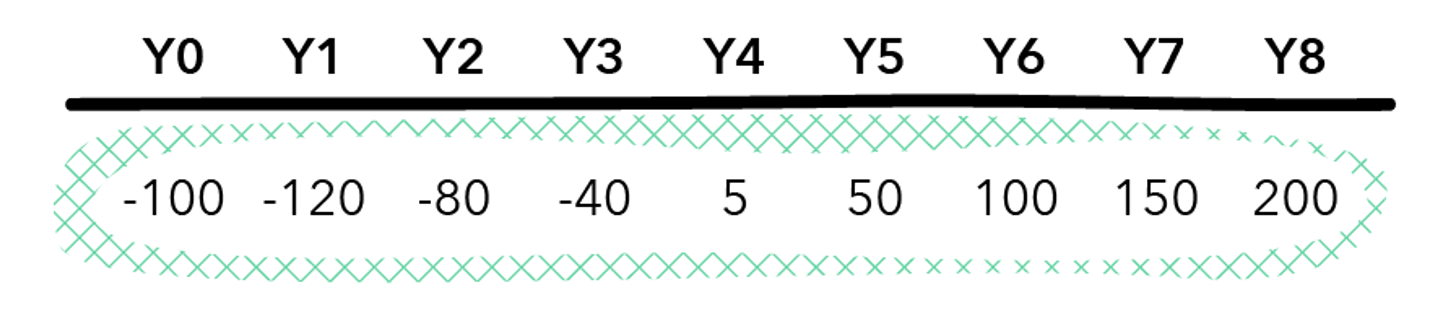Now, let’s take a look at the NPV of these cashflows using various discount rates.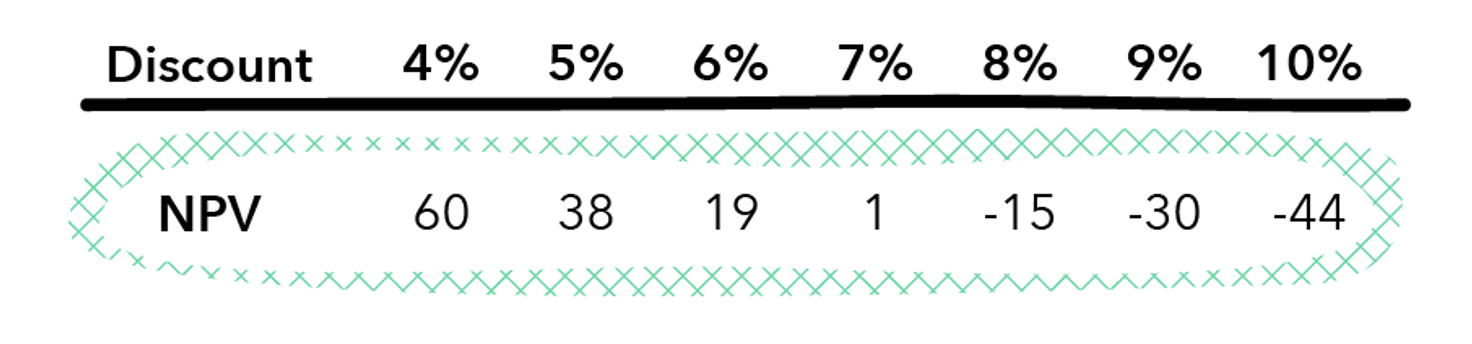As you would expect, the higher the discount rate (i.e. the higher the minimum required return), the lower the net present value of the cash flows to an investor.

In between 7% and 8%, we see that the NPV crosses over from positive to negative. The IRR is somewhere in between these numbers. While difficult to calculate manually, a financial calculator, or the Excel IRR function, will tell you that the number is 7.07%.

In general, the higher the IRR, the better. However, the golden rule to remember is that the IRR should be higher than the WACC

You should not use the IRR in isolation. For example, picking the project with the ‘highest IRR’ out of three potential options may not make sense if all are NPV negative (i.e. if even the highest IRR is lower than the WACC), or if each project has a different risk profile and debt-equity mix (and hence a different WACC).

### Profitability Index (PI)

The Profitability Index is another way of expressing how much a project’s NPV exceeds its initial investment. It is like the IRR, but instead of a percentage, it is expressed as a ratio.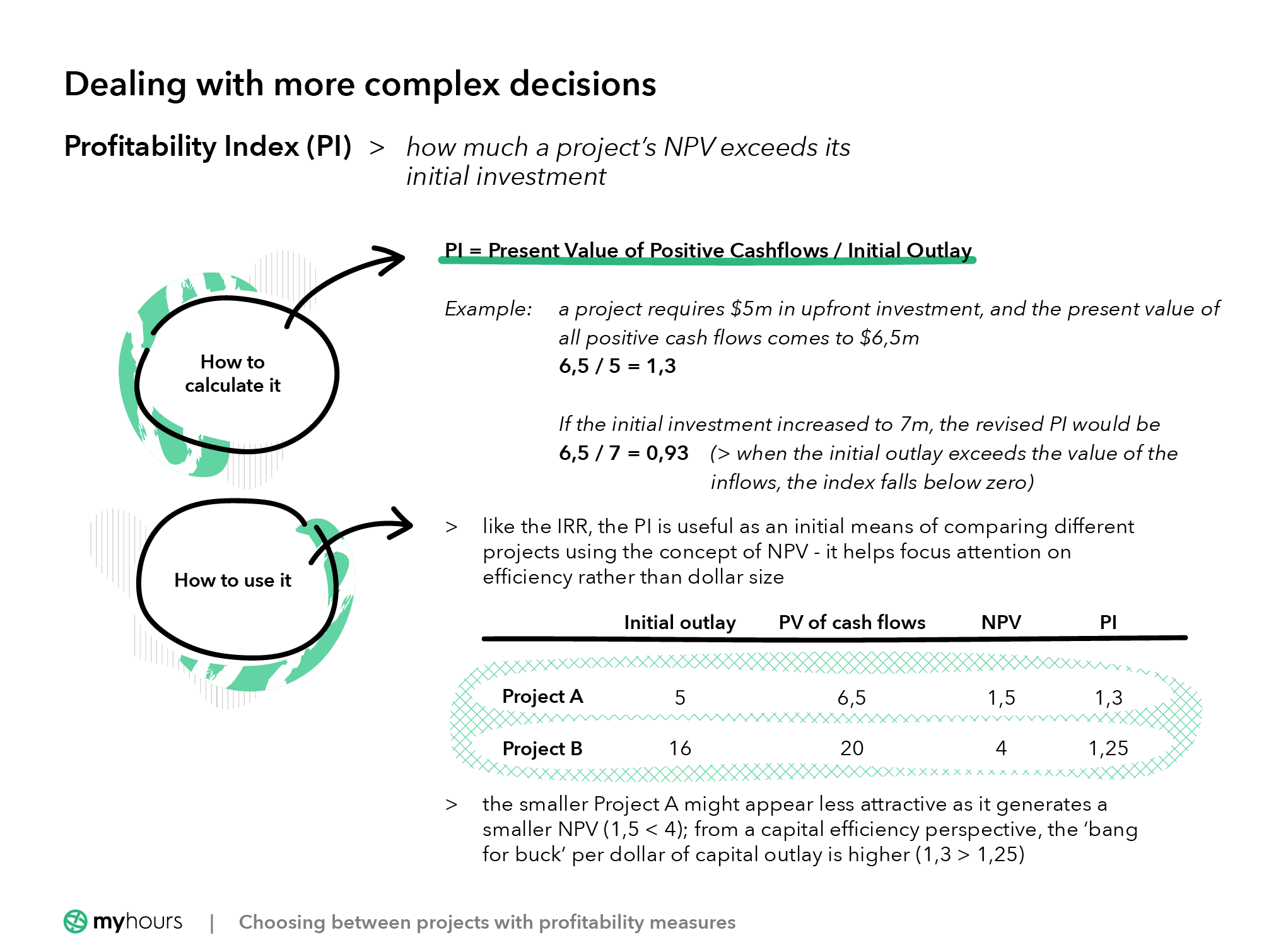#### How to calculate it

It works by separating out the positive cash flows (the project returns) from the negative cash flows (the initial investment). The calculation is as follows:

PI = Present Value of Positive Cashflows / Initial Outlay

#### How to use it

To take an example, suppose our project requires \$5m in upfront investment, and the present value of all positive cash flows comes to \$6.5m. The PI for this project would be:

6.5 / 5 = 1.3

Suppose the initial investment increased to 7m, the revised PI would be:

6.5 / 7 = 0.93

In other words, when the initial outlay exceeds the value of the inflows (i.e. NPV is negative), the index falls below zero.

Using the index figure instead of NPV allows us to normalize for project size, as in the table below: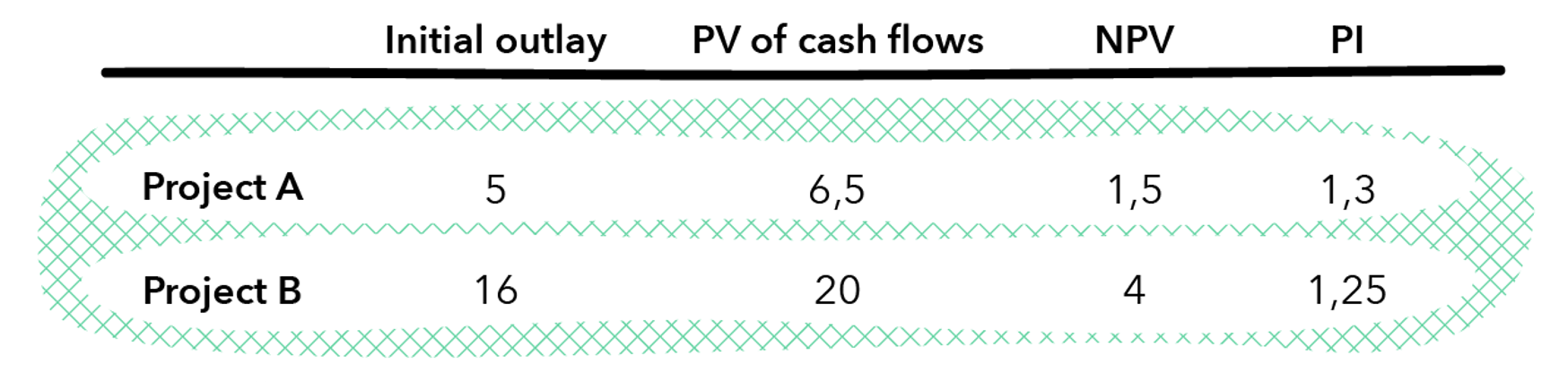The smaller Project A might appear less attractive as it generates a smaller NPV (1.5 < 4). From a capital efficiency perspective, the ‘bang for buck’ per dollar of capital outlay is higher (1.3 > 1.25).

Like the IRR, the PI is useful as an initial means of comparing different projects using the concept of NPV. It helps focus attention on efficiency rather than dollar size.

## Conclusion

As you would expect, each of the methods described above has advantages and ideal use cases. Ultimately, there is no substitute for careful modeling and good assumptions. An over-reliance on rules of thumb will trip you up in the end.

The process of modeling cash flows is not to predict the future (which is impossible), but to ensure that you go into projects with your eyes open.

Good luck!

←  Back to Time Tracking Library